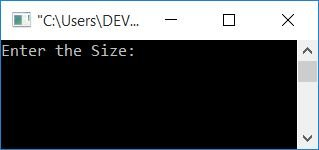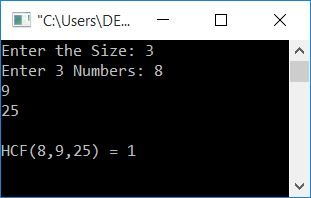# C Program to Find the HCF of n Numbers

In this article, you will learn and get code for finding the HCF of n numbers. However, before you begin the program, I recommend that you first visit Find HCF of Two Numbers (if you haven't already). Because the program given here is related to the program in that article.

## In C, find the HCF of n numbers

Now let's create a program to find the HCF of all the numbers given by the user at run-time.

```#include<stdio.h>
#include<conio.h>
int main()
{
int arr, n, mp, i, count;
printf("Enter the Size: ");
scanf("%d", &n);
printf("Enter %d Numbers: ", n);
for(i=0; i<n; i++)
scanf("%d", &arr[i]);
i=0;
mp = arr[i];
while(i<n)
{
if(mp<arr[i])
mp = arr[i];
i++;
}
while(1)
{
i=0;
count=0;
while(i<n)
{
if(arr[i]%mp==0)
count++;
i++;
}
if(count==n)
break;
else
mp--;
}
printf("\nHCF(");
for(i=0; i<(n-1); i++)
printf("%d,", arr[i]);
printf("%d) = %d", arr[i], mp);
getch();
return 0;
}```

This program was built and runs in the Code::Blocks IDE. Here is the sample run:Now supply the size, say 3, and enter any 3 numbers, say 8, 9, and 25. Press the ENTER key to see the following output:Here is another sample run for two numbers:This program is identical to the previous one, LCM of n Numbers in C. Except that we've switched the condition of the if block from:

`mp%arr[i]==0`

to

`arr[i]%mp==0`

and the statement from:

`mp++;`

to

`mp--;`

Why these two are reversed is discussed in LCM and HCF of Two Numbers in C. The brief description is:

• In the case of LCM, we have to find multiple (lowest common)
• In the case of HCF, we have to find factor (highest common)

And because multiples of a number are greater than the number, factors of a number are less than the number. I hope you are getting some idea about it. If not, then kindly visit:

C Quiz

« Previous Program Next Program »